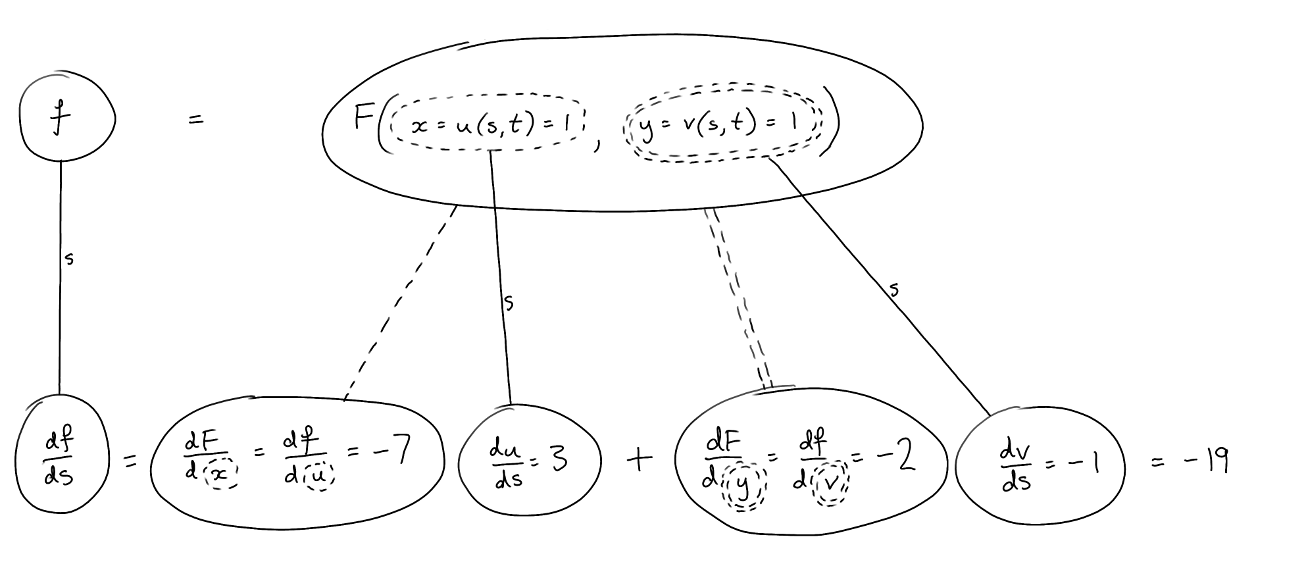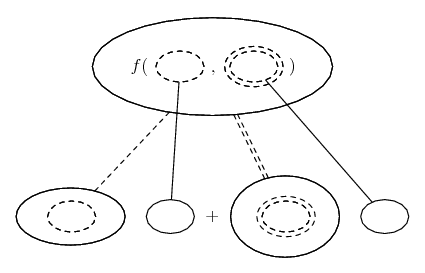# Thread: Partial differentiation question

1. ## Partial differentiation question

I am trying to do the first part of #12. The answer is -19.

I'm trying things like:
∂f/∂s = ∂F/∂u = ∂F/∂x * ∂x/∂u + ∂F/∂y * ∂y/∂u
but then I am stuck.

Could someone please show me how to do this properly?

Any help would be greatly appreciated!

2. ## Re: Partial differentiation question

Just in case a picture helps...... where (key in spoiler) ...

Spoiler:is the chain rule for two inner functions, i.e...

$\displaystyle \frac{d}{dx}\ f(u(x), v(x)) = \frac{\partial f}{\partial u} \frac{du}{dx} + \frac{\partial f}{\partial v} \frac{dv}{dx}$

Or rather, in this example...

$\displaystyle \frac{d}{ds}\ f(x(s), y(s)) = \frac{\partial f}{\partial x} \frac{dx}{ds} + \frac{\partial f}{\partial y} \frac{dy}{ds}$

As with...... the ordinary chain rule, straight continuous lines differentiate downwards (integrate up) with respect to s, and the straight dashed line similarly but with respect to the (corresponding) dashed balloon expression which is (one of) the inner function(s) of the composite expression.

We need a similar (double) diagram to differentiate with respect to t.

You can simplify the picture by losing many of the labels. Indeed you might have just values in most of the balloons.

Then do similar for dt, where the continuous lines mean with respect to t, and s is held constant.

Then do similar for the second derivatives.

_________________________________________

Don't integrate - balloontegrate!

Balloon Calculus; standard integrals, derivatives and methods

Balloon Calculus Drawing with LaTeX and Asymptote!

#### Search Tags

differentiation, partial, question